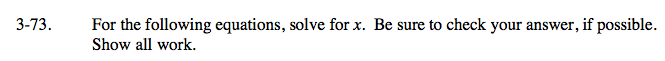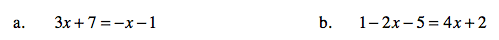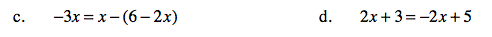### Home > CAAC > Chapter 3 > Lesson 3.2.1 > Problem3-73

3-73.Subtract 7 from both sides.

3x + 7 = −x − 1
− 7 − 7

Get the variable on a side by itself.

3x = −x − 8
+x +x

4x = −8

x = −2

Follow the steps in part (a).Follow the steps in part (a).

How does the negative sign in front of the parentheses change what is inside?

Follow the steps in part (a).

x = 0.5